# Free Convective Oldroyd Fluid Flow through an Annulus under Transverse Magnetic Field Using Modified Bessel Functions

Free Convective Oldroyd Fluid Flow through an Annulus under Transverse Magnetic Field Using Modified Bessel Functions

Debasish Dey Ardhendu Sekhar Khound

Department of Mathematics, Dibrugarh University, Dibrugarh 786004, Assam, India

Corresponding Author Email:
debasish41092@gmail.com
Page:
41-47
|
DOI:
https://doi.org/10.18280/ijht.370105
3 March 2018
|
Accepted:
11 July 2018
|
Published:
31 March 2019
| Citation

OPEN ACCESS

Abstract:

An unsteady visco-elastic fluid flow through an annulus with heat and mass transfer has been studied. The visco-elastic fluid is characterized by Oldroyd fluid constitutive model consisting of rheological parameters namely relaxation time (λ1) and retardation time (λ2). The annulus is bounded by two infinite co-axial circular cylinde

Keywords:

relaxation and retardion, visco-elastic fluid, modified Bessel function, shearing stress, annulus, radiation

1. Introduction

In recent time, visco-elastic fluid flow has attracted many scientists and researchers because of its uses in various industries such as polymer solution, suspension, paints, cosmetic products etc. Oldroyd [1, 2] has proposed the model of Oldroyd fluid to study flow pattern of visco-elastic fluid and its constitutive equation is given by

$σ_{ij}=-pδ_{ij}+τ_{ij}$ &

$(1+λ_1\frac{d}{dt})τ_{ij}=2μ(1+λ_2\frac{d}{dt})e_{ij}$ (1)

where, σij = stress tensor, p = Kronecker delta, τij = viscous-stress tensor, λ1 = relaxation time, λ2 = retardation time, μ= co-efficient of viscosity, eij = strain tensor and $\frac{d}{dt}$ = material derivative.

Messiha  has formulated the flow problem of laminar boundary layer past an infinite flat plate with variable suction. Cogley et al.  have analyzed the differential approximation for radiative heat transfer in a non-gray gas near equilibrium. Free convective heat transfer of the incompressible fluid flow restricted among a long vertical wavy wall and parallel flat wall has been investigated by Vajravelu and Sastri . Ray et al.  have evaluated exact periodical explanation of Oldroyd fluid governed by magnetic field in a channel. Chamkha  has carried out the solution of time dependent hydro-magnetic flow with thermal diffusion in channels and circular pipes. Hayat et al.  have analyzed some simple flow problems using Oldroyd-B fluid model. Hydro-magnetic Couette flow of Oldroyd-B fluid in rotating system has been investigated by Hayat et al. . Hayat et al.  have analyzed the Hall current effect on the flows of Oldroyd-B fluid through porous medium using cylindrical geometries. MHD oscillatory Couette flow with radiative heat diffusion in a porous medium with periodic wall temperature has been investigated by Israel-Cookey et al. . Biswas and Chakraborty  have investigated the pulsatile blood flow through catheterized artery with an axially nonsymmetrical stenosis. Pires and Sequeira  have studied the flow of generalized Oldroyd B fluids in curved pipe. Some exact solutions of Oldroyd-B fluid flows due to time dependent shear stresses have been analyzed by Jamil et al. . Shahid et al.  have carried out the exact solution of Oldroyd-B fluid flow over an infinite flat plate with oscillating shear stress. Singh  have analyzed the exact solution of hydro-magnetic flow problem with mixed convection through a rotating vertical channel with heat radiation. Deka and Paul  have investigated the nature of transient free convective MHD flow past an infinite vertical cylinder. Ahmed and Dutta  have studied the effects of radiative thermal diffusion in a time dependent MHD flow through an infinite annulus.  Time dependent thin film flow of Oldroyd-B fluid above an oscillating inclined belt under the influence of magnetic field have been analyzed by Gul et al. .

Application of visco-elastic fluid flow is seen in the blood circulatory system; some parts of energy are stored due to elastic nature of blood and another part is dissipated to heat due to viscosity and the remaining part is used for the movement of blood . Dey and Khound  have investigated the relaxation and retardation effects on free convective visco-elastic fluid flow past an oscillating plate and the governing equations of motion are solved analytically using perturbation scheme. Hall current effects on the binary mixture flow of Oldroyd-B fluid through a porous channel have been analyzed using separation of variable technique by Dey and Khound . Dey  has formulated the solution of the visco-elastic fluid flow problem through an annulus with relaxation, retardation effects and external heat source/sink. In our work, we have extended the work of  by introducing the mass transfer effects.

2. Mathematical formulations

The unsteady free convective hydro-magnetic Oldroyd fluid flow through the annular region formed by two infinite co-axial circular cylinders has been considered. In this problem, we have used the cylindrical polar co-ordinate system (r,θ,z). The flow is taken along the z-axis (axis of annulus), so the radial and cross radial velocity components are zero (u'=v'=0) and the assumption of axial symmetry states that the motion is independent of θ.  Further, we have assumed that the pressure gradient is varying periodically with time. The magnetic field of uniform strength B0 is applied perpendicular to the axis of annulus. Body forces generated during the fluid motion are Lorentz force (result of applied magnetic field) and buoyancy force (leads to the free convection). All fluidic properties are considered as constant, except the density variation in the buoyancy term. Energy dissipation due to viscosity is neglected. Induced magnetic field is neglected as the magnetic Reynolds number is very small for weekly conducting system. Rate of radiative heat transfer in optically thin fluid is given by term 4I(T'-Ts) (Cogley et al. ). The geometry of the problem is given by figure 1.

## 1.png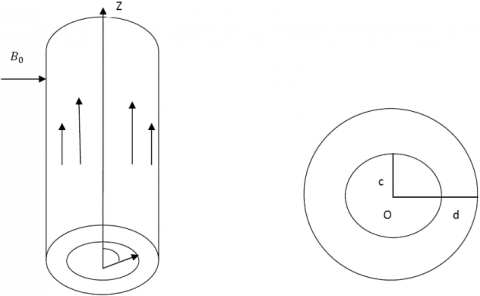Figure 1. Geometry of the problem 

From continuity equation, w'=w' (r,t)

Using the above assumptions, the governing equations of fluid motion are:

$\frac{∂w'}{∂t'}=-[\frac{1}{ρ} \frac{∂p'}{∂z'}+g]+\frac{1}{ρ}(\frac{∂}{∂r'}+\frac{1}{r'})τ'_{rz}-\frac{σB_0^2w'}{ρ}$ (2)

$\frac{∂T'}{∂t'}=\frac{K}{ρC_p}(\frac{∂^2T'}{∂r'^2 }+\frac{1}{r'}\frac{∂T'}{∂r'})-\frac{4I}{ρC_p}(T'-T_s )+\frac{Q_0}{ρC_p}(T'-T_s)$ (3)

$\frac{∂C'}{∂t'}=D(\frac{∂^2 C'}{∂r'^2}+\frac{1}{r'}\frac{∂C'}{∂r'})-k_1(C'-C_s )$ (4)

and the constitutive equation is given by

$(1+ λ_1\frac{∂}{∂t'})τ'_{rz}=μ(1+ λ_2\frac{∂}{∂t'}) \frac{∂w'}{∂r'}$ (5)

In static case, equation (2) reduces to $0=-[\frac{∂p_s}{∂z'}+ρ_s g]$, where ρs density of static fluid and ps is static fluid pressure. Then from (2), we get

$\frac{∂w'}{∂t'}=-[\frac{1}{ρ} \frac{∂(p'-p_s)}{∂z'}+\frac{(ρ-ρ_s)}{ρ} g]+\frac{1}{ρ} (\frac{∂}/{∂r'}+\frac{1}{r'}) τ'_{rz}-\frac{σB_0^2w'}{ρ}$ (6)

The equation of state following  is given by,

$ρ_s=ρ[1+β(T'-T_s )+β^* (C'-C_s )]$ (7)

Using (5), (6) and (7), we get

$\frac{∂w'}{∂t'}$$+λ_1\frac{∂^2w'}{∂t'^2}$$=-(1+λ_1\frac{∂}{∂t'})\frac{σB_0^2 w'}{ρ}+ν{\frac{∂^2w'}{∂r'^2}+\frac{1}{r}\frac{∂w'}{∂r'}+λ_2(\frac{∂^3w'}{∂r'^2∂t'}+\frac{1}{r}\frac{∂^2 w'}{∂r'∂t'}}-\frac{1}{ρ}(1+λ_1\frac{∂}{∂t'})\frac{∂p*}{∂z'}+(1+λ_1\frac{∂}{∂t'})(gβ(T'-T_s)+gβ*(C'-C_s ))$ (8)

where, p*=p'-ps. The boundary conditions of the problem are:

w'=0 at r=c & w'=0 at r=d

T'=Ts+ Ts n1 eiω't'  at r=c &

T'=Ts+ Ts n2 eiω't'  at r=d

C'=Cs+ Cs m1eiω't'  at r=c &

C'=Cs+ Csm2eiω't'  at r=d.

3. Method of solution

To convert the equations (8), (3) and (4) into dimensionless forms, we have introduced the following dimensionless quantities:

$w=\frac{w'c}{ν}, r=\frac{r'}{c}, z=\frac{z'}{c}, p=\frac{p^*c^2}{μυ}, t=\frac{t'ν}{c^2}$,

$λ=\frac{d}{c}, ψ=\frac{T'-T_s}{T_s} ,ϕ=\frac{C'-C_s}{C_s} ,a=\frac{λ_1 ν}{c^2}$

$b=\frac{λ_2 ν}{c^2} , M=\frac{σB_0^2c^2}{μ}, Gr=\frac{gβT_s ν^2}{c^3}$ ,

$Gm=\frac{gβ^* C_sν^2}{c^3}, N=\frac{4Ic^2}{μC_p}, h_c=\frac{k_1c^2}{D}$

$Qr=\frac{Q_0c^2}{μC_p}, Pr=\frac{μC_p}{K},Sc=\frac{ν}{D}, τ=\frac{τ'}{ρ\frac{ν^2}{c^2}}$ ,

and the non-dimensional equations are:

$\frac{∂w}{∂t}+a\frac{∂^2 w}{∂t^2}=-(1+a\frac{∂}{∂t})[Mw+\frac{1}{ρ} \frac{∂p^*}{∂z}-Grψ-Gmϕ]+(1+b\frac{∂}{∂t})(\frac{∂^2 w}{∂r^2}+\frac{1}{r} \frac{∂w}{∂r})$ (9)

$\frac{∂ψ}{∂t}=\frac{1}{Pr} (\frac{∂^2 ψ}{(∂r^2})+\frac{1}{r} \frac{∂ψ}{∂r})+(Qr-N)ψ$ (10)

$Sc\frac{∂ϕ}{∂t}=(\frac{∂^2ϕ}{∂r^2}+\frac{1}{r} \frac{∂ϕ}{∂r})+h_c ϕ$ (11)

The relevant boundary conditions are

w=0 at r=1,   w=0 at r=λ

ψ=n1eiωt  at r=1,  ψ=n2eiωt  at r=λ,

ϕ=m1eiωt  at r=1,  ϕ=m2eiωt  at r=λ

where, w is dimensionless velocity, r and z are dimensionless displacement variables, p = pressure, t = dimensionless time, λ = non zero constant, ψ = dimensionless temperature, ϕ = dimensionless concentration, a = dimensionless relaxation parameter, b = dimensionless retardation parameter, M = magnetic parameter, Gr = Grashoff number for heat transfer, Gm = Grashoff number for mass transfer, N = radiation parameter, Qr = heat source/ sink parameter, Pr = Prandtl number, Sc = Schmidt number, hc = chemical reaction parameter.

To solve the equation (10), we assume that $ψ=f(r) e^{iωt}$, then we get

$f''+\frac{1}{r} f'-η^2 f=0$ (12)

where, prime denotes the differentiation with respect to r. To solve the equation (9), we follow the method of complex variable used by Messiha , Vajravelu and Sastri  and Ahmed and Dutta. The periodic pressure gradient is given as follows :

$-\frac{∂p}{∂z}=P_0 e^{iωt}$

Let s=irη and $f(r)=f(\frac{s}{iη})=f_1(s)$, then (12) becomes

${s^2f_1}''+s{f_1}'+s^2 f_1=0$ (13)

Equation (13) is a Bessel equation and its solution is given by:

$f(r)=A_1 I_0 (rη)+B_1[-\frac{2}{π} K_0 (rη)-\frac{1}{i} I_0(rη)]$ (14)

Now, to solve the equation (11), we assume that ϕ=f^*(r)e^{iωt}, then we get

${f^*}''+\frac{1}{r}{f^*}'-ξ^2 f^*=0$ (15)

Let s*=irξ and $f^* (r)=f^* (\frac{s^*}{iξ})=f^*_1(s^* )$, then (15) becomes

${s^{*2}{f^*}_1}''+s^*{{f^*}_1}'+s^{*2}{f^*}_1=0$ (16)

Equation (16) is a Bessel equation and its solution is given by:

$f^* (r)=A_2 I_0 (rξ)+B_2 [-\frac{2}{π}K_0 (rξ)-\frac{1}{i} I_0 (rξ)]$ (17)

To solve the equation (9), we assume that $w=g(r)e^{iωt}, h=irγ, g(r)=g(\frac{h}{iγ})=g_1(h)$  then we get

$g''+\frac{1}{r}g'-γ^2g=D_3+iD_4+(D_5+iD_6 )f(r)+(D_7+iD_8 ) f^*(r)$ (18)

Using variation of parameter technique, the equation is solved and the solution in terms of following constants

$η^2=N-Qr+iω,ξ^2=Sciω+h_c,γ^2=D_1+iD_2$

$B_1=\frac{(n_2 I_0(η)-n_1I_0(λη)}{\frac{-2}{π}{[K_0(λη)I_0(λ)-K_0(η)I_0(λη)]}}'$,

$A_1=\frac{n_1+B_1{\frac{2}{π}K_0(η)+\frac{1}{i}I_0(η)}}{(I_0(η)}$,

$B_2=(m_2 I_0 (ξ)-m_1 I_0 (λξ))/((-2)/π [K_0 (λξ) I_0 (ξ)-K_0 (ξ) I_0 (λξ)] )$,

$A_2=(m_1+B_2 {2/π K_0 (ξ)+1/i I_0 (ξ)})/(I_0 (ξ) )$,

$D_1=((M-aω^2 )+(aM+1)bω^2)/(1+b^2 ω^2 )$,

$D_2=(b(aω^2-M)ω+(aM+1)ω)/(1+b^2 ω^2 )$,

$D_3=-(P_0+aP_0 bω^2)/(1+b^2 ω^2 )$,

$D_4=(〖bP〗_0 ω-aP_0 bω)/(1+b^2 ω^2 ), D_5=-Gr (1+abω^2)/(1+b^2 ω^2 )$,

$D_6=-Gr (a-b)ω/(1+b^2 ω^2 ),D_7=-Gm (1+abω^2)/(1+b^2 ω^2 ), D_8=-Gm (a-b)ω/(1+b^2 ω^2 )$

is given by

## eq_191.png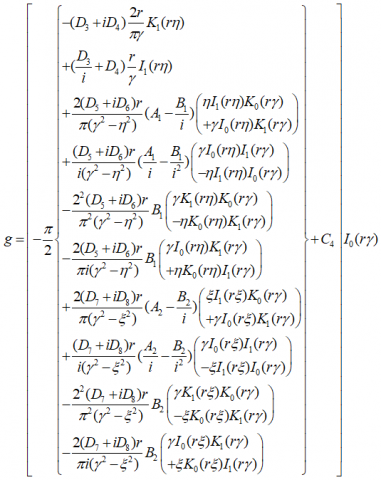## eq_192.pngThe constants of the above solutions are given below.

4. Results and discussions

To get the exact solutions, analytical method has been used. After solving the equations using Bessel equations, finally MATLAB software have been used to get the numerical values for plotting the graphs.

The dimensionless shearing stress of the fluid motion is expressed in the form of first order linear differential equation given as follows:

$(1+a \frac{∂}{∂t})τ=(1+b\frac{∂}{∂t})\frac{∂w}{∂r}$

The shearing stress at the inner cylinder is given by

$τe^\frac{t}{a}=L_1a(\frac{1+ibω}{1+iaω}){{e^(\frac{1}{a}+iω)t-1}}$

Figure 2 to 6 represent the periodical shearing stress against the time variable for various values of flow parameters involved in the solution. It is seen that during the increment of Prandtl number by 66.67 % (from Pr=3 to Pr=5), the shearing stress increases by 60 % (approximately) [Figure 2], it can be interpreted that the Prandtl number (ratio of viscosity to thermal diffusivity) enhances the viscosity of the fluid and as a consequence, shear stress (directly proportional to viscosity of the medium) on the wall increases.

## 2.pngFigure 2. Shearing stress at the lower surface against time t for ω=0.5, Sc=2, hc=3.5, N=0.1, Qr=0.4, λ=0.8, r=1.6, n1=0.1, n2=0.2, m1=0.03, m2=0.4, a=0.3, b=0.2, Gr=2, Gm=3, M=2, P0=0.1

## 3.png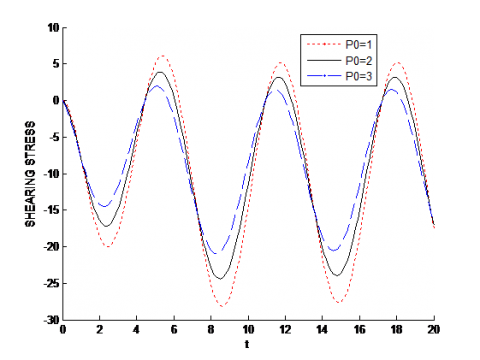Figure 3. Shearing stress at the lower surface against time t for ω=0.5, Sc=2, Pr=5, hc=3.5, N=0.1, Qr=0.4, λ=0.8, r=1.6, n1=0.1, n2=0.2, m1=0.03, m2=0.4, a=0.3, b=0.2, Gr=2, Gm=3, M=2

## 4.png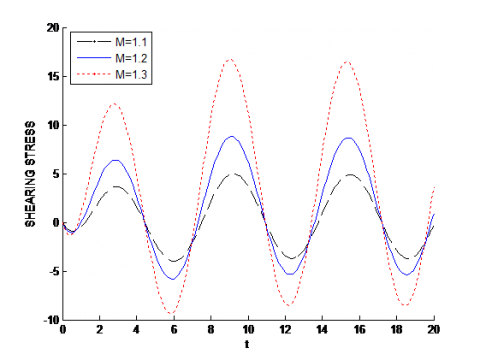Figure 4. Shearing stress at the lower surface against time t for ω=0.5, Sc=2, Pr=5, hc=3.5, N=0.1, Qr=0.4,

0.8, r=1.6, n1=0.1, n2=0.2, m1=0.03, m2=0.4, a=0.3, b=0.2, Gr=2, Gm=3, P0=0.1

Figure 3 represents the variation of shearing stress for various values of pressure gradient. It is noticed that fluid flow experiences lesser viscous drag at the surface during the enhancement of pressure gradient. This encourages the researchers that to reduce the shear stress, the pressure gradient may be increased. Statistically, it is seen that there is a decline trend in shearing stress possibly by 71.43 % during the growth in pressure gradient by 200 %.

In general, the Lorentz force has a resistive impact on the fluid motion and it increases the shearing stress. This physical phenomenon is matched with the result obtained from figure 4. Statistically, it is seen that the shearing stress significantly by almost 200 % for 18 % enhancement in magnetic parameter [Figure 4].

Grashoff numbers generally characterize the free convective properties of fluid motion. It is seen that the magnitude of shearing stress is increased during the increase of Grashof number for heat transfer [Figure 5] but an opposite trend is observed for increasing values of Grashof number for mass transfer [Figure 6].

## 5.pngFigure 5. Shearing stress at the lower surface against time t for ω=0.5, Sc=2, Pr=5, hc=3.5, N=0.1, Qr=0.4, λ=0.8, r=1.6, n1=0.1, n2=0.2, m1=0.03, m2=0.4, a=0.3, b=0.2, Gm=3, M=2, P0=0.1

## 6.pngFigure 6. Shearing stress at the lower surface against time t for ω=0.5, Sc=2, Pr=5, hc=3.5, N=0.1, Qr=0.4, λ=0.8, r=1.6, n1=0.1, n2=0.2, m1=0.03, m2=0.4, a=0.3, b=0.2, Gr=2, M=2, P0=0.1

## 7.pngFigure 7. Temperature against time t for ω=0.5, Sc=2, Pr=5, hc=3.5, Qr=0.4, λ=0.8, r=1.6, n1=0.1, n2=0.2, m1=0.03, m2=0.4, a=0.3, b=0.2, Gr=2, Gm=3, M=2, P0=0.1

## 8.pngFigure 8. Temperature against time t for ω=0.5, Sc=2, hc=3.5, N=0.1, Qr=0.4, λ=0.8, r=1.6, n1=0.1, n2=0.2, m1=0.03, m2=0.4, a=0.3, b=0.2, Gr=2, Gm=3, M=2, P0=0.1

## 9.png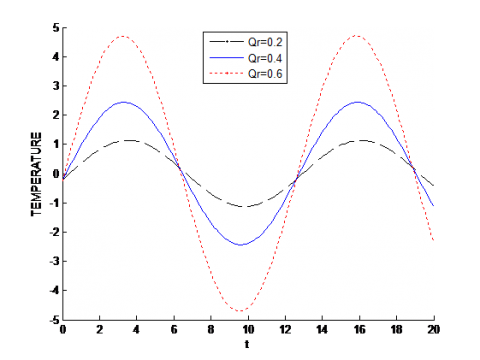Figure 9. Temperature against time t for ω=0.5, Sc=2, Pr=5, hc=3.5, N=0.1, λ=0.8, r=1.6, n1=0.1, n2=0.2, m1=0.03, m2=0.4, a=0.3, b=0.2, Gr=2, Gm=3, M=2, P0=0.1

## 10.pngFigure 10. Concentration against time t for ω=0.5, Sc=2, Pr=5, N=0.1, Qr=0.4, λ=0.8, r=1.6, n1=0.1, n2=0.2, m1=0.03, m2=0.4, a=0.3, b=0.2, Gr=2, Gm=3, M=2, P0=0.1

## 11.pngFigure 11. Concentration against time t for ω=0.5, Pr=5, hc=3.5, N=0.1, Qr=0.4, λ=0.8, r=1.6, n1=0.1, n2=0.2, m1=0.03, m2=0.4, a=0.3, b=0.2, Gr=2, Gm=3, M=2, P0=0.1

Figure 7-9 depict the periodical variation of temperature profiles against the time for various flow parameters. Radiation is a mode of heat transfer, i.e., there is a heat flow with the higher values of radiation. As a result, the net temperature of the system declines. This physical phenomenon is seen in figure 7.

Figure 8 states that during the enhancement of Prandtl number, there is an increment of temperature. Physically, it may be interpreted that due to higher Prandtl number, the frictional force (form of viscosity) increases and as a result, temperature enhances.

Effects of heat source/sink parameter (Qr) on temperature profile is shown by Figure 9. Qr>0 characterizes heat source and Qr<0 characterizes heat sink. There is significant enhancement in temperature during external heat source agent. Statistically, it is observed that the temperature increases by 345.45 % (approximately) during 200% rise in Qr.

Figures 10 & 11 portray the periodical nature of concentration profiles against time for various flow parameters. It is also revealed that there is significant increment in concentration profile during the increment in chemical reaction parameter (Figure 10) and Schmidt number (Figure 11). Physically it is possible because, the chemical reaction parameter and Schmidt number are inversely proportional to the molecular diffusion rate and as a consequence the concentration of the fluid increases.

5. Conclusions

From the above study, the following points are concluded

• Fluid dynamics have been used extensively in various industries. Lots of industrial works such as chemical mixing devices, bearings, drilling of oil wells etc. are based on fluid flow through a cylindrical pipe or between two concentric circular pipes. The presence of friction might damage the surface. So, to overcome this, shearing stress formed at the surface must be in control. Shearing stress on the surface can be controlled by increasing the pressure gradient and Grashoff number for mass transfer or by reducing the Prandtl number, magnetic parameter, Grashoff number for heat transfer
• By increasing the radiation parameter the temperature of the system may be reduced.
• Prandtl number and heat source have a positive impact on temperature.
• Concentration of the fluid is increased with the increase of chemical reaction parameter and Schmidt number.
6. Future scope

The present work of free convective Oldroyd fluid flow through an annulus with applied magnetic field has been done using Bessel functions. In future, this work may be extended into following directions:

• To cover all hydro-magnetic aspects, induced magnetic field may be included.
• Suction at the both the walls may be taken for broader applications in engineering
• Suitable numerical methods may be used and then it may be compared with the results obtained by analytical methods.
• Some other fluid models like micro-polar fluid, nano fluid etc. may be used in place of Oldroyd fluid.
Nomenclature

w'=velocity of fluid at time t', p'=pressure, p*=p'-ps, ρ=density of fluid, T'=temperature of fluid, Ts=temperature of fluid at static case, C' =concentration of fluid, Cs=concentration of the fluid at static case, ν=kinematics viscosity, g =acceleration due to gravity, β=co-efficient of volume expansion for heat transfer, β*=co-efficient of volume expansion for mass transfer, B0=strength of the magnetic field,  σ=electrical conductivity of the fluid, c=radius of inner cylinder, d=radius of outer cylinder (d>c), Cp=specific heat at constant pressure, τ'rz=viscous stress, D=molecular diffusivity, k1=chemical reaction parameter, and n1, n2, m1, m2 are non-zero constants, w is dimensionless velocity, r and z are dimensionless displacement variables, p=pressure, t=dimensionless time, λ=non zero constant, ψ=dimensionless temperature, ϕ =dimensionless concentration, a =dimensionless relaxation parameter, b =dimensionless retardation parameter, M=magnetic parameter, Gr=Grashoff number for heat transfer, Gm=Grashoff number for mass transfer, N=radiation parameter, Qr=heat source/sink parameter, Pr=Prandtl number, Sc=Schmidt number, hc=chemical reaction parameter.

References

 Oldroyd JG. (1950). On the formulation of rheological equations of state. Proceedings of the Royal Society of London, Series A, Mathematical and Physical Sciences 200(1063): 523–541. https://doi.org/10.1098/rspa.1950.0035

 Oldroyd JG. (1958). Non-Newtonian effects in steady motion of some idealized elastico-viscous liquids. Proceedings of Royal Society of London A245: 278-297. https://doi.org/10.1098/rspa.1958.0083

 Messiha SAS. (1966). Laminar boundary layers in oscillatory flow along an infinite flat plate with variable suction. Math. Proc. Camb. Philos. Soc. 62(2): 329-337. https://doi.org/10.1017/S030500410003989X

 Cogley AC, Gilles SE, Vincenti WG. (1968). Differential approximation for radiative transfer in a nongrey gas near equilibrium. AIAA Journal 6(3): 551-553. https://doi.org/10.2514/3.4538

 Vajravelu K, Sastri KS. (1978). Free convective heat transfer in a viscous incompressible fluid confined between a long vertical wavy wall and a parallel flat wall. Journal of Fluid Mechanics 86(2): 365-383. https://doi.org/10.1017/S0022112078001172

 Ray RN, Samad A, Chaudhury TK. (1999). An exact periodic solution of hydromagnetic flow of an Oldroyd fluid in a channel. ASME Journal of Applied Mechanics 66: 974-977. https://doi.org/10.1115/1.2791807

 Chamkha AJ. (2000). Unsteady laminar hydromagnetic fluid-particle flow and heat transfer in channels and circular pipes. International Journal Heat and Fluid Flow 21(6): 740-746. https://doi.org/10.1016/S0142-727X(00)00031-X

 Hayat T, Siddiqui AM, Asghar S. (2001). Some simple flows of an Oldroyd-B fluid. International Journal of Engineering Science 39: 135-147. https://doi.org/10.1016/S0020-7225(00)00026-4

 Hayat T, Nadeem S, Asghar S. (2004). Hydromagnetic Couette flow of an Oldroyd-B fluid in a rotating system. International Journal of Engineering Science 42: 65–78. https://doi.org/10.1016/S0020-7225(03)00277-5

 Hayat T, Hussain M, Khan M. (2006). Hall effect on flows of an Oldroyd-B fluid through porous medium for cylindrical geometries. Computers and Mathematics with Applications 52: 269-282. https://doi.org/10.1016/j.camwa.2006.08.018

 Israel-Cookey C, Amos E, Nwaigwe C. (2010). MHD oscillatory Couette flow of a radiating viscous fluid in a porous medium with periodic wall temperature. American Journal of Scientific and Industrial Research 1(2): 326-331. https://doi.org/2010.1.2.326.331

 Biswas D, Chakraborty US. (2010). Pulsatile blood flow through a Catheterized artery with an axially no symmetrical stenosis. Appl. Math. Science 4(58): 2865-2880.

 Pires M, Sequeira A. (2011). Flow of generalized Oldroyd B fluids in curved pipe. Progress in Non-linear Differential Equations and their Applications 80: 21–4. https://doi.org/10.1007/978-3-0348-0075-4_2

 Jamil M, Fetecau C, Rana M. (2012). Some exact solutions for Oldroyd-B fluid due to time dependent prescribed shear stress. Journal of Theoretical Applied Mechanics 50(2): 549-562.

 Shahid N, Rana M, Siddique I. ( 2012). Exact solution for motion of an Oldroyd-B fluid over an infinite flat plate that applies an oscillating shear stress to the fluid. Boundary Value Problems 48: 1–19. https://doi.org/10.1186/1687-2770-2012-48

 Singh KD. (2013). Exact solution of MHD mixed convection periodic flow in a rotating vertical channel with heat radiation. International Journal of Applied Mechanics and Engineering 18(3): 853-869. https://doi.org/10.2478/ijame-2013-0051

 Deka RK, Paul A. (2013). Transient free convective MHD flow past an infinite vertical cylinder. Theoretical Applied Mechanics 40(3): 385-402. https://doi.org/10.2298/TAM1303385D

 Ahmed N, Dutta M. (2015). Heat transfer in an unsteady MHD flow through an infinite annulus with radiation. Boundary Value Problems 2015(1): 1-17. https://doi.org/10.1186/s13661-014-0279-z

 Gul T, Islam S, Shah RA, Khalid A, Khan I, Shafie S. (2015). Unsteady MHD thin film flow of an Oldroyd-B fluid over an oscillating inclined belt. PLOS 10(7): 1-18. https://doi.org/10.1371/journal.pone.0126698

 Hemorheology. Wikipedia. https://en.wikipedia.org/wiki/Hemorheology#Blood_viscoelasticity. Accessed on 27 August 2016.

 Dey D, Khound AS. (2016). Relaxation and retardation effects on free convective visco-elastic fluid flow past an oscillating plate. International Journal of Computer Applications 144(9): 34-40. https://doi.org/10.5120/ijca2016910434

 Dey D, Khound AS. (2016). Hall current effects on binary mixture flow of Oldroyd-B fluid through a porous channel. International Journal of Heat and Technology 34(4): 687-693. https://doi.org/10.18280/ijht.340419

 Dey D. (2017). Viscoelastic fluid flow through an annulus with relaxation, retardation effects and external heat source/sink. Alexandria Engineering Journal 1-7. https://doi.org/10.1016/j.aej.2017.01.039